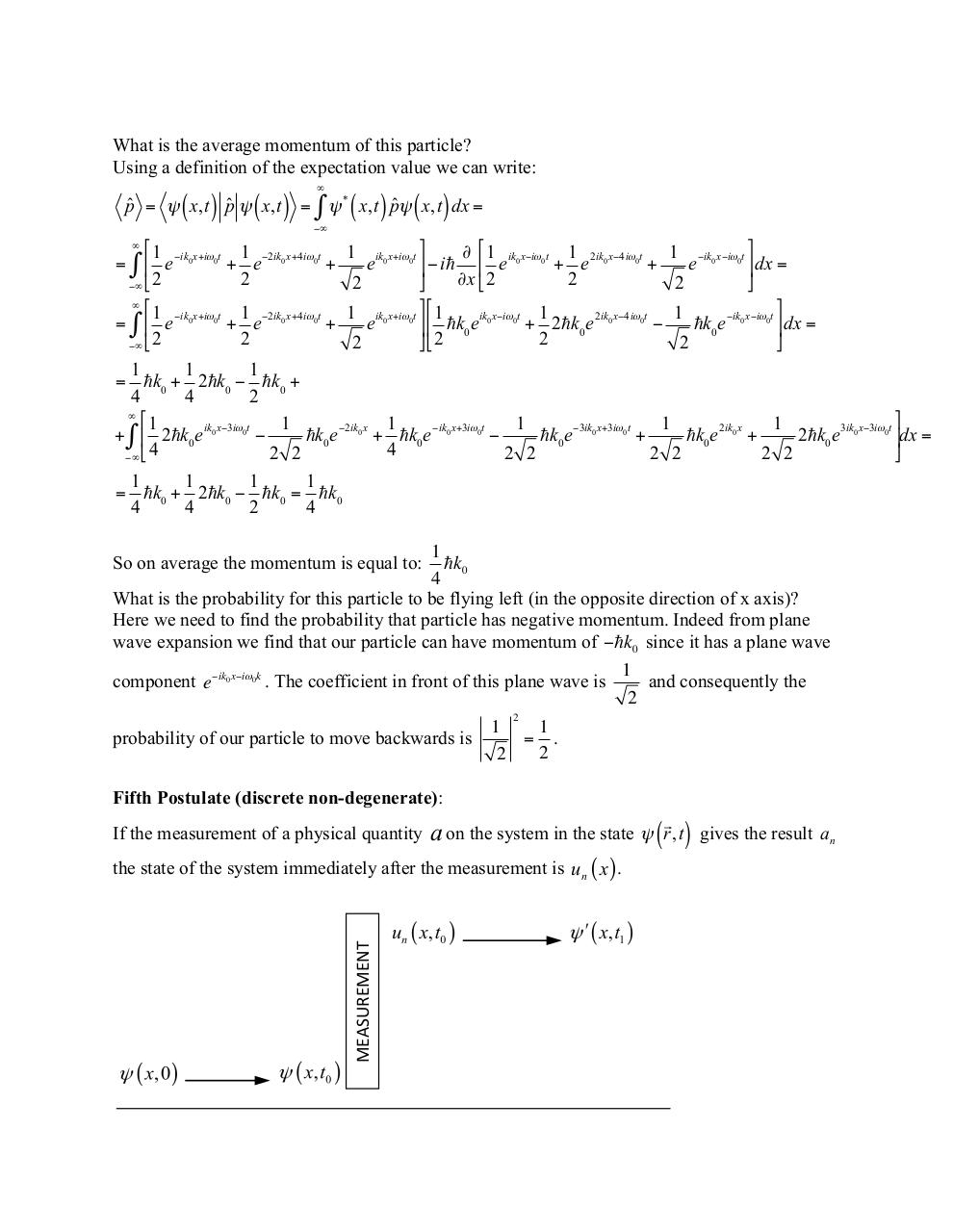# L7+QM+Measurements+and+Constants+of+Motion.pdfPage 1 2 3 4 5 6 7 8 9

#### Text preview

What is the average momentum of this particle?
Using a definition of the expectation value we can write:

pˆ = ψ x,t pˆ ψ x,t = ∫ ψ * x,t pˆψ x,t dx =

( )

( )

−∞

∞\$
1 −ik x+iω t 1 −2ik x+4iω t
= ∫&amp; e 0 0 + e 0 0 +
2
−∞ % 2
∞\$
1 −ik x+iω t 1 −2ik x+4iω t
= ∫&amp; e 0 0 + e 0 0 +
2
−∞ % 2

( ) ( )

'
∂ \$ 1 ik x−iω t 1 2ik x−4iω t
) − i &amp; e 0 0 + e 0 0 +
∂x % 2
2
(
2
1 ik0 x+iω0t '\$ 1
1
ik x−iω t
2ik x−4iω t
e
)&amp; k0e 0 0 + 2k0e 0 0 −
2
(% 2
2
1

e

ik0 x+iω0t

'
) dx =
(
2
'
1
−ik x−iω t
k0e 0 0 ) dx =
(
2

1

e

−ik0 x−iω0t

1
1
1
= k0 + 2k0 − k0 +
4
4
2
∞\$
'
1
1
1
1
1
1
ik x−3iω t
−2ik x
−ik x+3iω t
−3ik x+3iω t
2ik x
3ik x−3iω t
+ ∫ &amp; 2k0e 0 0 −
k0e 0 + k0e 0 0 −
k0e 0 0 +
k0e 0 +
2k0e 0 0 )dx =
4
(
2 2
2 2
2 2
2 2
−∞ % 4
1
1
1
1
= k0 + 2k0 − k0 = k0
4
4
2
4
1
k0
4
What is the probability for this particle to be flying left (in the opposite direction of x axis)?
Here we need to find the probability that particle has negative momentum. Indeed from plane
wave expansion we find that our particle can have momentum of −k0 since it has a plane wave
1
component e−ik0 x−iω0k . The coefficient in front of this plane wave is
and consequently the
2
So on average the momentum is equal to:

2

probability of our particle to move backwards is

1
1
= .
2
2

Fifth Postulate (discrete non-degenerate):

a on the system in the state ψ ( r,t )
the state of the system immediately after the measurement is un ( x ) .
MEASUREMENT

If the measurement of a physical quantity

gives the result an